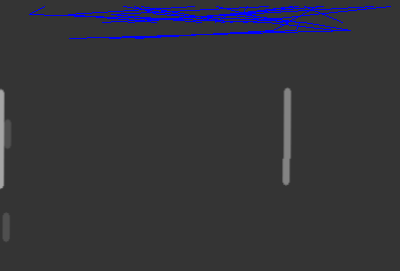# Debugging PTQ | ResNet

Hi, I’m looking for suggestions on ways to debug the quantization steps.

I’ve a model architecture with ResNet-18 backbone, a neck and a head. Made the required changes to ResNet BasicBlock to make it quantizable.

``````lass BasicBlock(nn.Module):
expansion = 1

def __init__(self,
inplanes,
planes,
stride=1,
downsample=None,
groups=1,
base_width=64,
dilation=1,
norm_layer=None):
super(BasicBlock, self).__init__()
if norm_layer is None:
norm_layer = nn.BatchNorm2d
if groups != 1 or base_width != 64:
raise ValueError(
'BasicBlock only supports groups=1 and base_width=64')
# if dilation > 1:
#     raise NotImplementedError(
#         "Dilation > 1 not supported in BasicBlock")
# Both self.conv1 and self.downsample layers downsample the input when stride != 1
self.conv1 = conv3x3(inplanes, planes, stride, dilation=dilation)
self.bn1 = norm_layer(planes)
# self.relu = nn.ReLU(inplace=True) # org
self.relu1 = nn.ReLU(inplace=True) # quantization mod
self.conv2 = conv3x3(planes, planes, dilation=dilation)
self.bn2 = norm_layer(planes)
self.downsample = downsample
self.stride = stride

# quantization mod
self.relu2 = nn.ReLU(inplace=True)

def forward(self, x):
identity = x

out = self.conv1(x)
out = self.bn1(out)
out = self.relu1(out)
out = self.conv2(out)
out = self.bn2(out)
if self.downsample is not None:
identity = self.downsample(x)

# Use FloatFunctional for addition for quantization compatibility
# out += identity
out = self.relu2(out)

return out
``````

Trying to quantize the model using the FX Graph approach. Here’s the code for the same

``````        device = "cpu"
self.net.to(device)
self.net = self.net.module.to(device)
self.net.eval()
data = next(it)
output = self.net(data)
input("model forward pass ok")

model_to_quantize = copy.deepcopy(self.net)

qconfig = get_default_qconfig("qnnpack")
qconfig_dict = {"": qconfig}

model_prepared = quantize_fx.prepare_fx(model_to_quantize, qconfig_dict)

if i%100==0:
print(i)
model(data['img'])

model_quantized = quantize_fx.convert_fx(model_prepared)

print_size_of_model(self.net)
print_size_of_model(model_quantized)

``````

The fp32 model is about 47Mb and the quantized one is about 12Mb, which is great. However, the outputs from quantized model is rubbish haha. Happy to hear thoughts on what could be going wrong.

Additionally, I’m looking at the quantized model layers (see below). Shouldn’t it be QuantizedConvBnReLU2d instead of QuantizedConvReLU2d?(QuantizedConv2dBn instead of QuantizedConv2d). Where can I find source code for these?``````   (backbone): Module(
(model): Module(
(conv1): QuantizedConvReLU2d(3, 64, kernel_size=(7, 7), stride=(2, 2), scale=0.017876433208584785, zero_point=0, padding=(3, 3))
(maxpool): MaxPool2d(kernel_size=3, stride=2, padding=1, dilation=1, ceil_mode=False)
(layer1): Module(
(0): Module(
(conv1): QuantizedConvReLU2d(64, 64, kernel_size=(3, 3), stride=(1, 1), scale=0.007517809513956308, zero_point=0, padding=(1, 1))
(conv2): QuantizedConv2d(64, 64, kernel_size=(3, 3), stride=(1, 1), scale=0.03266022354364395, zero_point=167, padding=(1, 1))
)
(1): Module(
(conv1): QuantizedConvReLU2d(64, 64, kernel_size=(3, 3), stride=(1, 1), scale=0.013314908370375633, zero_point=0, padding=(1, 1))
(conv2): QuantizedConv2d(64, 64, kernel_size=(3, 3), stride=(1, 1), scale=0.048815514892339706, zero_point=169, padding=(1, 1))
)
)
``````

One of the things I’m considering is to just quantize the ResNet backbone. Appreciate leads in the form of blogs, repos on this. Thanks!

Edit:

specifically, I created a synthetic dataset and am trying to get the model to detect lines. With a float32 model, I get good detections (which is reflected in recall and other performance numbers). But with a quantized model, the outputs are as shown below.for accuracy problem I think one thing you can try is to explicitly set the quantized engine to qnnpack: Quantization — PyTorch 1.13 documentation

for conv bn issue, bn is fused into conv in PTQ

@jerryzh168 , thanks for your response.

I switched to quantizing the model in Eager Mode, it seems to work.

For my understanding, do I need to add QuantStub and DequantStub while quantizing via FX Graph mode?

In Eager mode, the user has to add quant/dequant modules.

In FX graph mode, the workflow will automatically add quant/dequant modules. As long as your model is symbolically traceable with FX, we recommend this workflow because it is less manual work.